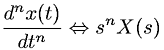Equations > Signal Processing > Laplace Transform Properties > Laplace transform Nth time domain derivative property

### Laplace transform Nth time domain derivative propertyLatex Code:

MathML Code:

 $\frac{d}^{n}x\left(t\right){\mathrm{dt}}^{n}⇔{s}^{n}X\left(s\right)$

MathType 5.0: